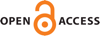Download this articleFor screen For printingRecent IssuesThe Journal About the Journal Editorial Board Editorial Interests Subscriptions Submission Guidelines Submission Page Policies for Authors Ethics Statement ISSN (electronic): 1472-2739 ISSN (print): 1472-2747 Author Index To Appear Other MSP JournalsAsymptotic translation lengths and normal generation for pseudo-Anosov monodromies of fibered $3$–manifolds

### Hyungryul Baik, Eiko Kin, Hyunshik Shin and Chenxi Wu

Algebraic & Geometric Topology 23 (2023) 1363–1398##### Abstract

Let $M$ be a hyperbolic fibered $3$–manifold. We study properties of sequences $\left({S}_{{\alpha }_{n}},{\psi }_{{\alpha }_{n}}\right)$ of fibers and monodromies for primitive integral classes in the fibered cone of $M$. The main object is the asymptotic translation length ${\ell }_{\mathsc{𝒞}}\left({\psi }_{{\alpha }_{n}}\right)$ of the pseudo-Anosov monodromy ${\psi }_{{\alpha }_{n}}$ on the curve complex. We first show that there exists a constant $C>0$ depending only on the fibered cone such that for any primitive integral class $\left(S,\psi \right)$ in the fibered cone, ${\ell }_{\mathsc{𝒞}}\left(\psi \right)$ is bounded from above by $C∕|\chi \left(S\right)|$. We also obtain a moral connection between ${\ell }_{\mathsc{𝒞}}\left(\psi \right)$ and the normal generating property of $\psi$ in the mapping class group on $S$. We show that for all but finitely many primitive integral classes $\left(S,\psi \right)$ in an arbitrary $2$–dimensional slice of the fibered cone, $\psi$ normally generates the mapping class group on $S$. In the second half of the paper, we study if it is possible to obtain a continuous extension of normalized asymptotic translation lengths on the curve complex as a function on the fibered face. An analogous question for normalized entropy has been answered affirmatively by Fried and the question for normalized asymptotic translation length on the arc complex in the fully punctured case has been answered negatively by Strenner. We show that such an extension in the case of the curve complex does not exist in general by explicit computation for sequences in the fibered cone of the magic manifold.

##### Keywords
asymptotic translation length, fibered 3–manifold, fibered cone, pseudo-Anosov, curve complex
##### Mathematical Subject Classification
Primary: 30F60, 37E30
Secondary: 32G15, 37B40
##### Publication